# Class 7 CBSE maths Integers

This page contains main concepts and results for Class 7, Chapter-1 INTEGERS. This chapter is mainly about representation of integers on the number line and their addition and subtraction.

## Numbers

We use numbers to count anything. So, what are various types of numbers?

### 1. Natural numbers

Natural numbers are counting numbers but these set of numbers do not include zero. This is because you cannot count zero. So numbers,
1,2,3,4,5,6……etc are all natural numbers

### 2. Whole numbers

All natural numbers along with zero are called whole number. For example
0, 1, 2, 3, 4, 5, 6………etc are all whole numbers.
NOTE:- Thes types of numbers do not include fractions.
From the definition of natural numbers we can conclude that every natural or counting number is a whole number.

## 3. Integers

Integers include all natural numbers, zero and negative numbers for example,
-4, -3, -2, -1, 0, 1, 2, 3, ………. etc are all integers.
So now we have,
1. Positive integers:- 1, 2, 3, ……
2. Negative integers:- -1, -2, -3, …….
3. 0 (zero):- which is an integer that is neither negative nor positive.
Note:- Integers like whole numbers do not include fractions for example 3.5 , ½ etc.

Number line for integers is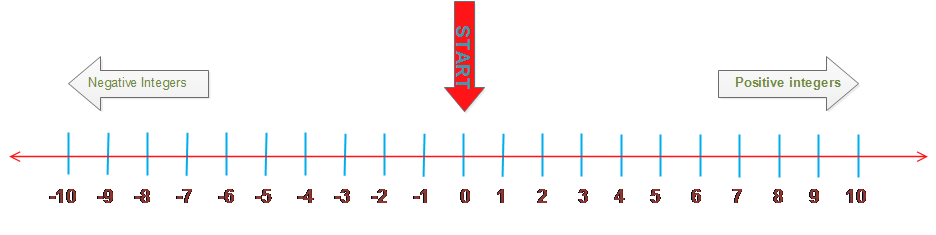Now from this number line you can see that numbers to the left of zero are all negative. Again from this number line you can observe that numbers to the right of zero are all positive.
Important note:- If the number has no sign attached to it as prefix then it means that it is a positive number.
For example number 3 is really number +3
Important Points
On the number line when we
1. Add a positive integer, we move to the right.
2. Add a negative integer we move to the left.
3. Subtract a positive integer we move to the left.
4. Subtract a negative integer, we move to the right.

## Properties of integers

• Integers are closed under addition, subtraction and multiplication. Which means that sum of integers will also give integers.
• Addition and multiplication are commutative for integers, i.e.,
1.  a + b = b + a
2. a × b = b × a
For any two integers a and b.
• Addition and multiplication are associative for integers, i.e.,
1. (a + b) + c =  a + (b + c)
2. (a × b) × c  =  a × (b × c)
for any three integers a, b and c.
• Existence of identity
1. Zero (0) is an additive identity for integers, i.e., a + 0 = 0 + a = a
for any integer a.
2.  1 is multiplicative identity for integers, i.e., a × 1 = 1 × a = a
for any integer a.
• Integers show distributive property of multiplication over addition, i.e., a × (b + c) = a × b + a × c
for any three integers a, b and c.
•  Product of a positive integer and a negative integer is a negative integer, i.e, a × (–b) = – ab
where a and b are positive integers.
• Product of two negative integers is a positive integer, i.e.,
• (–a) × (–b) = ab
where a and b are positive integers.
• Product of even number of negative integers is positive, whereas the product of odd number of negative integers is negative, i.e.,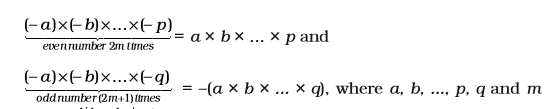• When a positive integer is divided by a negative integer or vice-versa and the quotient obtained is an integer then it is a negative integer, i.e., $a\div \left( b \right)=\left( a \right)\div b\text{ =}\frac{a}{b}$
where a and b are positive integers and $-\frac{a}{b}$is an integer
• When a negative integer is divided by another negative integer to give an integer then it gives a positive integer, i.e., $\left( a \right)\div \left( b \right)=\frac{a}{b}$ , where a and b are positive integers and $\frac{a}{b}$ is also an integer.
• For any integer a,   $a\div 1=a~$
and
a ÷ 0 is not defined.
• ## Rules of addition of integers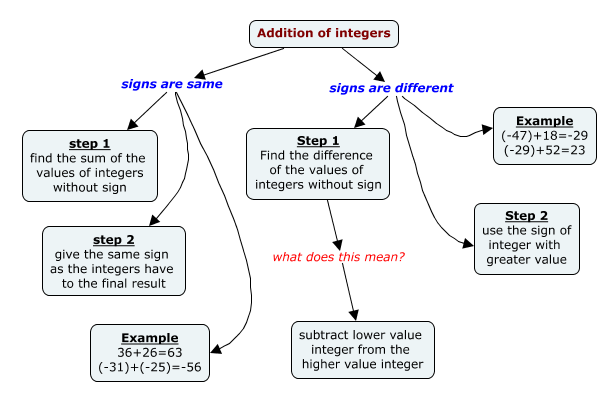## Rules for subtraction of integers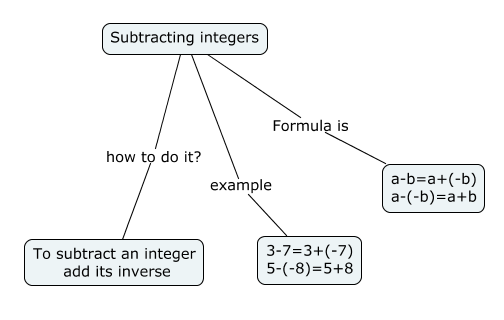## Rules for multiplication and division of integers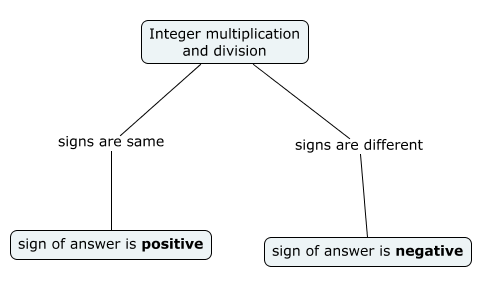## Problem solving strategy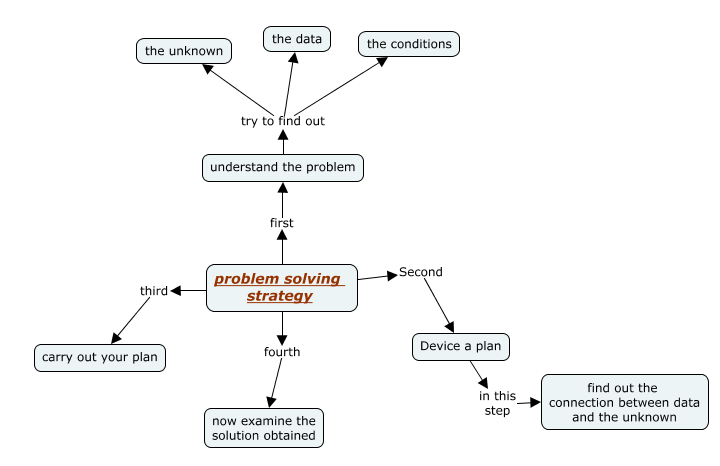Now let us consider solving a problem and apply the above mentioned problem solving strategy. For this purpose we will solve a NCERT book problem.
Question:
Mohan deposits Rs 2,000 in his bank account and withdraws Rs 1,642 from it, the next day. If withdrawal of amount from the account is represented by a negative integer, then how will you represent the amount deposited? Find the balance in Mohan's account after the withdrawal.
Solution:
Step 1:- Understand the problem For this step first read your problem carefully. So,
• What do you know from the problem?
Here find ‘what is the amount Mohan deposited in his bank account and how much he withdraws’.
• What are you trying to find?
Balance in Mohan’s bank account after the withdrawal.
Amount deposited = Rs 2000
Amount withdrawn = Rs 1642
Here we have to find the amount he removed from his account. So,
Balance in Mohan's account = Money deposited - Money withdrawn
Step 3:- Solve the problem
Now that you have all the known and unknown quantities and you also have a strategy to solve your problem, you can now carefully carry out your calculations.
Balance in Mohan's account = 2000 + (-1642) = 2000 - 1642 = 358
Therefore, balance in Mohan's account after withdrawal is Rs 358.
Step 4:- Revise